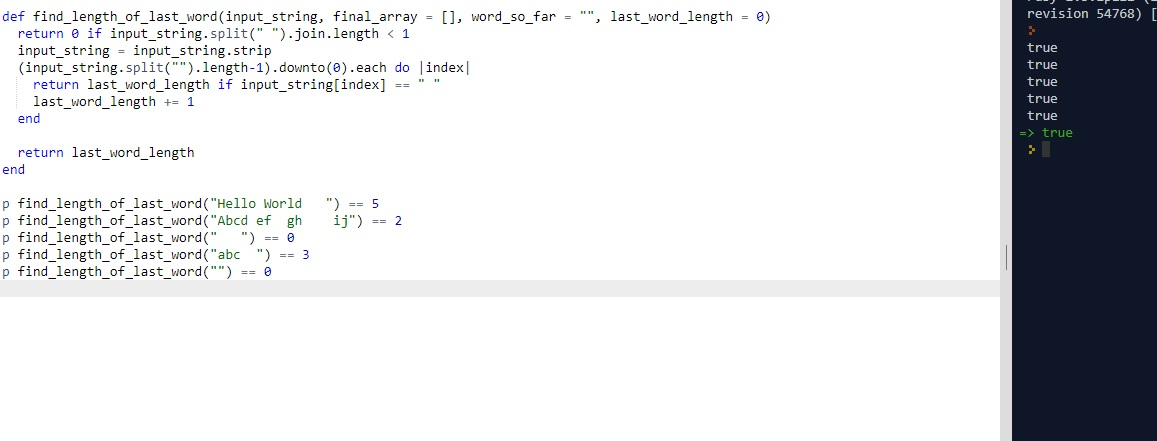# CLEAN CODE: LENGTH OF LAST WORD

By: Saurav

2017-11-09 07:32:00 UTC

Given a string s consists of upper/lower-case alphabets and empty space characters ' ', return the length of last word in the string.

If the last word does not exist, return 0.

Note: A word is defined as a character sequence consists of non-space characters only.

Example:

Given s = "Hello World",

return 5 as length("World") = 5.

Please make sure you try to solve this problem without using library functions. Make sure you only traverse the string once.

InterviewBit

Attempt 1:
First of all lets write some tests first:

```p find_length_of_last_word("Hello World") == 5
p find_length_of_last_word("Abcd ef  gh    ij") == 2
p find_length_of_last_word("") == 0
p find_length_of_last_word("abc  ") == 3
p find_length_of_last_word("   ") == 0```

My first idea was to use simple split function to get the job done.

```def find_length_of_last_word(input_string, final_array = [])
return 0 if input_string.strip.length < 1
return input_string.strip.split(" ")[-1].length
end

```

Note: A very important point is that str.strip returns a copy of string str with with whitespace chopped off, while str.strip! returns the same string str or nil if the string wasn't altered.

In the above case we used strip so there won't be nil for split which builds a chain of method calls on objects.

Attempt 2:

Notice the question says minimum use of library functions. Lets try one without.

My simple strategy is to :
1. Iterate through the string in reverse order.
2. If a space is found, return the word length so far
3. Or update word length by 1

Return word length

```def find_length_of_last_word(input_string, final_array = [], word_so_far = "", last_word_length = 0)
return 0 if input_string.split(" ").join.length < 1
input_string = input_string.strip
(input_string.split("").length-1).downto(0).each do |index|
return last_word_length if input_string[index] == " "
last_word_length += 1
end

return last_word_length
end ```Another option is to use strip (and not strip!) as the first filter.

```def find_length_of_last_word(input_string, final_array = [], word_so_far = "", last_word_length = 0)
input_string = input_string.strip
return 0 if input_string.length < 1

(input_string.split("").length-1).downto(0).each do |index|
return last_word_length if input_string[index] == " "
last_word_length += 1
end

return last_word_length
end ```

I like this one. Its more efficient and for average time complexity is much better since we are start from the end.

Notes:
1. (" ").strip will return the string ""
2. (" asas ").strip will strip the starting and ending white spaces. Return will be "asas"
3. (" as as ").strip does not strip away the white spaces in between characters. Return will be "as as"
4. ("").strip returns ""
5. ("").strip! will return nil, so don't use it if you want to build a chain of method calls on objects like str.strip.split(" ")[-1] etc

Let me know what you think or suggest! :)

Owned & Maintained by Saurav Prakash

If you like what you see, you can help me cover server costs or buy me a cup of coffee though donation :)